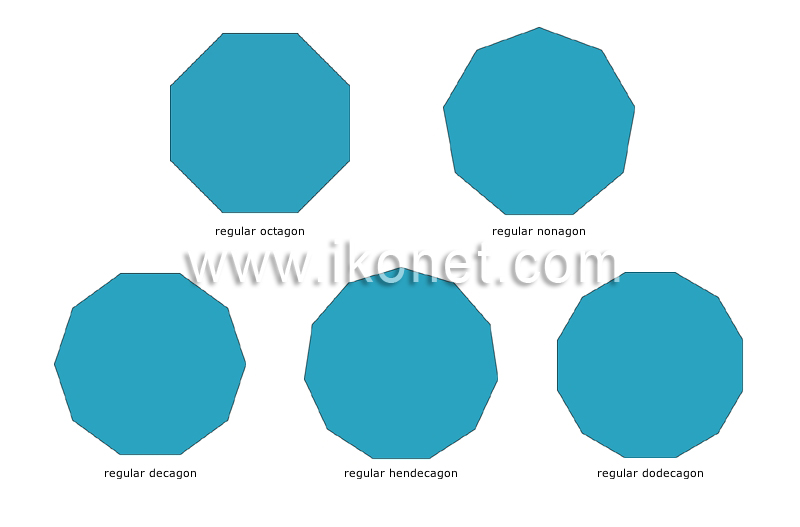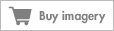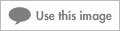Look up an image:

#### polygonsGeometric plane figures with several sides and a number of equal angles.See polygons in : french | spanish

#### regular hendecagonPolygon with 11 (hendeca = eleven) sides and equal angles.

#### regular dodecagonPolygon with 12 (dodeca = twelve) sides and equal angles.

#### regular decagonPolygon with 10 (deca = ten) sides and equal angles.

#### regular nonagonPolygon with nine (nona = nine) sides and equal angles.

#### regular octagonPolygon with eight (octo = eight) sides and equal angles.